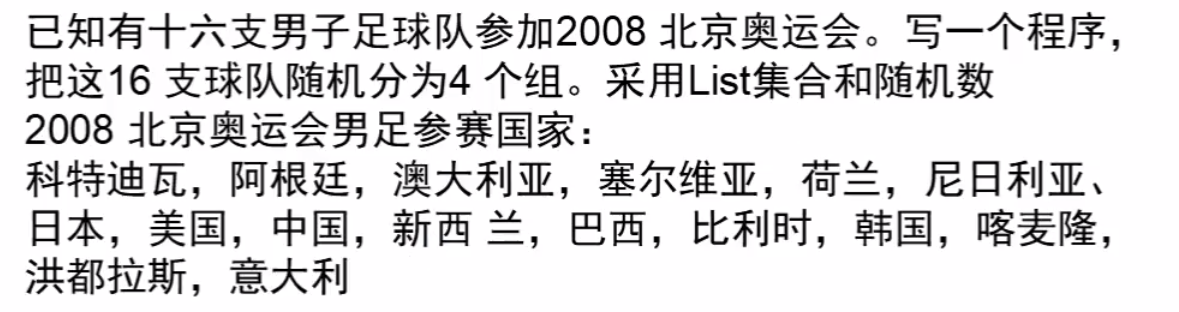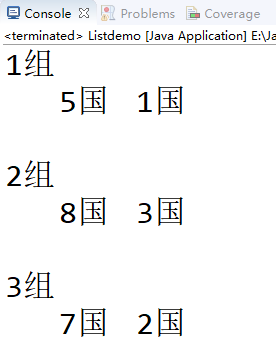# Java作业把这16支球队随机分为4个组,采用List集合和随机数• 首先创建个`List`集合并赋值

• 创建个随机数 `Random`

• 用 for 循环 第一层循环例如是分成4组就 <=4   再比如是3组就  <=3

• 第二层循环 是每组 要几个人！或者几个队！和上面一样的思路

• 第二层循环 里面 首先要获取下标  并删除这个 数  为了就下次不再循环到他！

`//随机获取一个 //a.size() 获取集合长度 //ran.nextInt(a.size()) 最大是这个长度`

``````package jihe;

import java.util.ArrayList;
import java.util.List;
import java.util.Random;

public class Listdemo {

public static void main(String[] args) {
//初始化 并赋值
List a = new ArrayList();

//生成随机数
Random ran = new Random();

for(int i =1;i<=3;i++){
System.out.println(i+"组");
for(int j = 0;j<2;j++){
//随机获取一个
//a.size() 获取集合长度
//ran.nextInt(a.size()) 最大是这个长度
Object b = a.get(ran.nextInt(a.size()));
//输出
System.out.print("   " + b);
//在集合中删除输出的
a.remove(b);
}
System.out.println("\n");

}

}

}``````